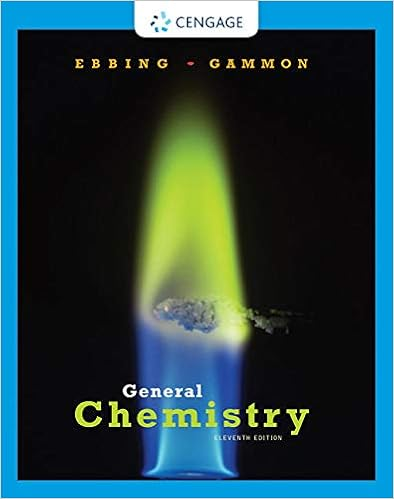# IA IN-Class 4-5 - Mat 108 Intermediate Algebra Unit 4 Part...

• Notes
• 12

This preview shows page 1 - 3 out of 12 pages.

##### We have textbook solutions for you!
The document you are viewing contains questions related to this textbook.The document you are viewing contains questions related to this textbook.
Chapter 1 / Exercise 1.92
General Chemistry
Ebbing/GammonExpert Verified
Mat 108 Intermediate AlgebraUnit 4 Part 5Equations that Lead to Quadratic EquationsThere are numerous examples of equations in mathematics that lead to quadratic equations; we will study two of these. The first type is rational equations. RATIONAL EQUATIONS IN ONE VARIABLEEXAMPLE: 212115525xxxRECOGNITION:1. The variable appears in some denominators2. There is an equal symbol3. There is only one variableWHAT DOES IT ASK?What replacements for “x” will make the valueof the expression 1255xxequalto the valueof the expression 21125x?OUTCOME: Usually the solution set will contain a finite number of solutions and they must be writteninside bracesSTRATEGY:As with any equation we encounter with fractions (denominators), the first step is toclear those denominators.To do this, we will find the LCD of all denominators on both sides of the equation and multiply both sides by the 1LCDto clear those denominators; however, this presents us with a mathematical dilemma. The Rules for equations say that “you can multiply both sides of an equation by any quantity except zeroand not affect the solution set”. The dilemma here is that the 1LCDusually involves the variableand we may be multiplying both sides by zeroand not know it. You will see how we make note of this in these examples. 1
##### We have textbook solutions for you!
The document you are viewing contains questions related to this textbook.The document you are viewing contains questions related to this textbook.
Chapter 1 / Exercise 1.92
General Chemistry
Ebbing/GammonExpert Verified
Here’s the basic strategy that we will apply to our examples: (1) Find the LCD of alldenominators in the whole problem; (2) Multiply both sidesby the 1LCDto clear the denominators; (3) Note the excluded (restricted) replacements(those replacements that would make the1LCDzero); (4) Solve the resulting equation; (5) Check your “answers” versus the excluded replacementsand write the solution set (inside braces). As you will see in the first example below, you may check you “answers” using the TI to verify that you have solved the problem correctly.Let’s try an example:Example 1:Solve 22203571234xxxxxThe first step is to factor all denominators and determine the LCD. The first denominatorconveniently factors as () ()34xxand the other two cannot be factored. Our LCD is () ()34xx. We will first multiply both sides by the () ()3411xxLCDorto clear the fractions; however, we mustlistour excluded replacements: 3, 4x(Is it obvious that 3 and 4 are the two replacements that would make () ()341xxequal to zero?) () ()() ()22343420351712314xxxxxxxxxProvided3, 4xMultiplying on each side can be very tricky – try to follow the steps here:2
•••